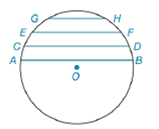Chapter 6.4, Problem 17EElementary Geometry For College St...

7th Edition
Alexander + 2 others
ISBN: 9781337614085

Solutions

Chapter
SectionElementary Geometry For College St...

7th Edition
Alexander + 2 others
ISBN: 9781337614085
Textbook Problem

Given parallel chords A B ¯ , C D ¯ , E F ¯ , and G H ¯ in circle O , which chord has the greatest length? Why?To determine

To find:

The chord that has the greatest length and the shortest length and the reason for that.

Explanation

Given:

The circle O and parallel chords AB¯, CD¯, EF¯, and GH¯.

The figure given below,

Theorem:

In a circle (or in congruent circles) containing two unequal minor arcs, the greater minor arc corresponds to the longer of the chords related to these arcs.

Calculation:

Using following theorem:

Since the arcs are unequal i.e

Still sussing out bartleby?

Check out a sample textbook solution.

See a sample solution

The Solution to Your Study Problems

Bartleby provides explanations to thousands of textbook problems written by our experts, many with advanced degrees!

Get Started

Evaluate each expression: 4+232

Elementary Technical Mathematics

6x2(3x2+2)+14(x+2)

Applied Calculus for the Managerial, Life, and Social Sciences: A Brief Approach

Differentiate the function. g(x)=74x23x+12

Single Variable Calculus: Early Transcendentals

True or False: f(x) = 3x x3 is concave down for x 1.

Study Guide for Stewart's Single Variable Calculus: Early Transcendentals, 8th